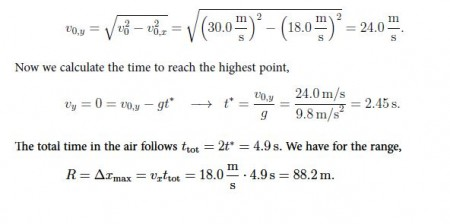# Motion in 2 Dimension: Mathematical Examples

Example 1: A projectile is launched with V0 = 19.2 m/s at angle θ0 = 38.7°. a) Does the projectile `clear’ a 3.0 m- high wall that is 30 m from the launch? b) How far behind the wall does the projectile hit the ground?

Solution: We calculate the components of the initial velocity vector:

Vx,0 = 19.2 m/s x cos 38.7° = 15 m/s

Vy,0 = 19.2 m/s x sin 38.7° = 12 m/s.

For this problem, we need to find the time when the projectile clears [or hits] the wall. That is, we set Δx = 30.0 m so that for the time t = t*:

t* =  Δx / Vx,0 = 30 m / 15 m/s = 2.0 s

We then find the vertical coordinate of the projectile at that instant,

y= 12 m/s x 2.0 s – ½ 9.81 m/s2 (2 s)2 = 24 m – 20 m = 4 m.

Since y > 3 m, the projectile clears the wall. The projectile falls to the ground when y = 0 so that

y = v0,y t — (g/2)t2 = (V0,y – gt/2)t = 2.45 s

We thus get for the horizontal displacement,

R = vx,0 t = 15 m/s x 2.45 s = 36.8 m.

That is, the projectile falls to the ground 6.8 m behind the wall.

Discussion: The time to reach the peak follows from vy = vy,0 — gt = 0 or tpeak = vy,0 /g . Thus, the time for the projectile to hit the ground is twice that of the projectile to reach the peak: this property reflects the symmetry of the trajectory.

The quantity R is referred to as the range. We find for the time to reach the peak Vpeak = v0 sin θ0 /g, so that for the range R = v0 cos θ0 x 2 v0 sin θ0 /g, or,

R = (v0 2 /g) sin2θ0

where we use 2 sin θ0 cos θ0 = sin 2θ0. This shows that the maximum range projectile is achieved when θ0 = 45°.

Example 2: A device is hidden inside a ball, enabling you to measure the speed of the ball but not its direction. You forget to write down the times at which the ball was flying with different speeds. The ball undergoes projectile motion in the Earth’s gravitational field. a) From your records, you determine that the maximum speed of the ball was 30.0 m/s, and that the minimum speed was 18.0 m/s. Find the direction in which the ball was launched. b) How long is the ball in air until it hits the ground? c) What distance along the ground does the ball travel through the air until it hits the ground?

Solution: We have vx = v0,x = vmin = 18.0 m/s. Since v0 = vmax = 30.0 m/s, we get:

V0,x =  v0  Cos θ0  or Cos θ0 = V0,x / V0 =  (18.0 m/s) / (30 m/s) = 0.6

For the angle, this gives 0o = 53.1° . We calculate the vertical component of the initial velocity: